GeeksforGeeks App
Open AppBrowser
Continue

## Related Articles

• CBSE Class 11 Physics Notes

# How to calculate Atmospheric Pressure?

Air being a fluid, exerts pressure at a point in a similar manner as a liquid column. The gaseous envelope surrounding the earth is called the atmosphere. And the pressure exerted by the atmosphere is called atmospheric pressure. The force exerted by the air column of air on a unit area of the earth surface is equal to the atmospheric pressure.

The atmospheric pressure at a point is equal to the weight of the column of air of unit cross-sectional area extending from that point to the top of the atmosphere.

In simple words, it is the force exerted on a surface by the air. The value of atmospheric pressure is 1.013 × 105 Pascal at sea level.

Some major units for measuring atmospheric pressure are as follows:

• 1 atm = 1.013 × 106 dyne/cm2

=  1.013 × 106 N/m2 (Pa)

• 1 bar = 106 dyne/cm2

= 105 N/m2

• 1 millibar (m bar) = 10-3 bar

= 103 dyne/cm2

= 102 N/m2

• 1 torr = 1 mm of Hg
• 1 atm = 101.3 kPa

= 1.013 bar

= 760 torr

### Devices to Measure the Atmospheric Pressure

Atmospheric pressure plays a vital role in determining the weather conditions and water chemistry etc. A rapid drop in the atmospheric pressure indicates a low-pressure system, this low-pressure system is mainly associated with rainy, cloudy weather. Whereas a rapid increase in the atmosphere pressure indicates clear sky and dry weather.

All these major changes are mainly measured using various devices to measure atmospheric pressure. These devices to measure atmospheric pressure have wide-ranging applications in our day-to-day lives. However, here are the five majorly used devices to measure atmospheric pressure are,

1. Mercury Barometer
2. Open-Tube Manometer
3. Hydraulic Machines
4. Hydraulic Lift
5. Hydraulic Brakes

### Mercury Barometer

An Italian scientist E. Torricelli was the first to devise a method for measuring atmospheric pressure accurately. Mercury Barometer is a simple instrument used to measure the atmospheric pressure at a point. It is a 1 m long glass tube, closed at one end is filled with clean and dry mercury. After closing the end of the tube with the thumb, the tube is inserted into a dish of mercury. As the thumb is removed, the mercury level in the tube falls down a little and comes to rest at a vertical height of 76 cm above the mercury level in the dish.

When it is then inverted over a trough containing mercury. Mercury in the tube slides down, till it becomes stable at a level. Due to this, the level of mercury rises in the trough. When the level of mercury become stable in the tube, we say, that the pressure exerted by mercury is the same as the atmospheric pressure. Let’s understand this with an example discussed below: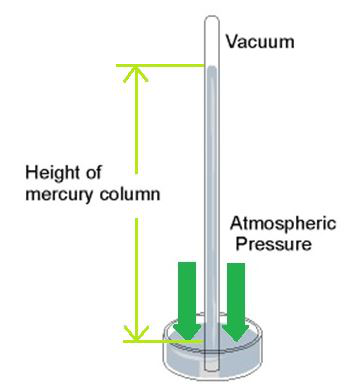Mercury Barometer

At sea level, the mercury column in the Barometer tube has a height of 76 m above the mercury level in the trough. Let us consider two points B and C at the same horizontal level, as shown in the figure. As they are at the same horizontal level the same pressure act at these points.

PC = PB = Pa

where Pa is the atmospheric pressure.

But according to the expression for absolute pressure,

PB = PA + ρgh

where ρ is the density of mercury, h is the height of mercury and PA is the pressure at the region above mercury inside the tube (= 0).

PC = PB = 0 + ρgh

PA = ρgh

At sea level Pa = 1 atm and h = 76 cm.

Therefore, 76 cm of mercury in a mercury barometer corresponds to the atmospheric pressure is equivalent to 1 atmosphere.

### Open-Tube Manometer

An open-tube manometer is an instrument used for measuring pressure differences, or to measure the pressure of gas enclosed in a vessel. It consists of a U-shaped tube open at both ends. A liquid of density ρ is filled in the tube. One end of the tube is left open to the atmosphere and the other end is connected to the system whose pressure is to be measured.

A suitable liquid is taken in the U-tube. To measure small pressure differences, we use a low-density liquid like oil in the manometer tube. Whereas to measure large pressure differences we use high-density liquids like mercury. Let’s understand this with an example discussed below: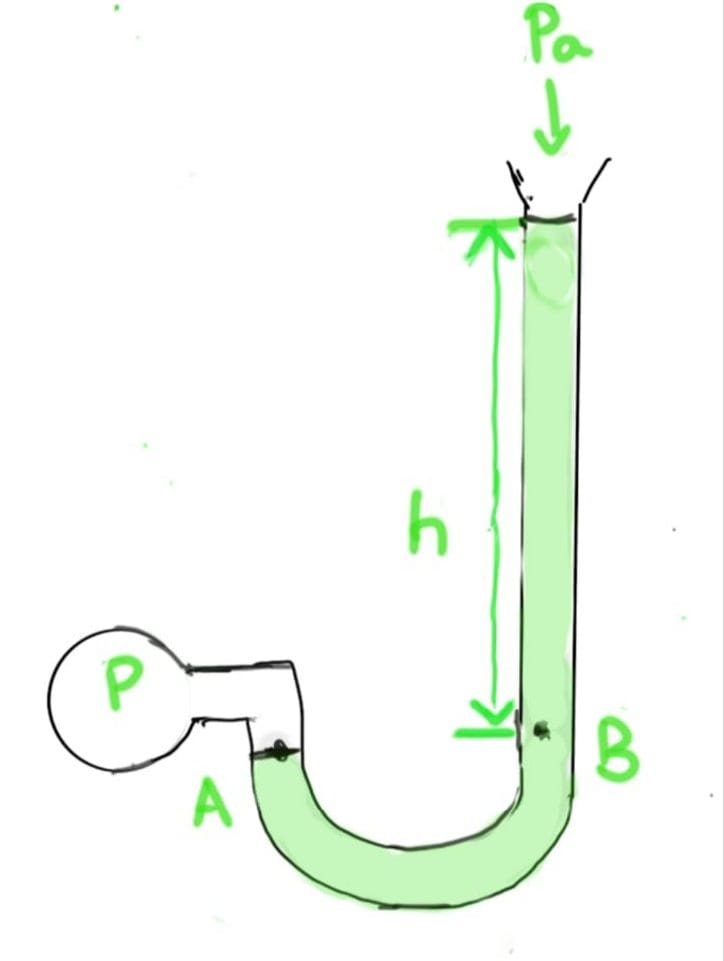Open-Tube Manometer

Let P be the pressure of the enclosed sample, and Pa be the atmospheric pressure. The liquid level in the open limb of the U-tube rises as compared to other limbs joined to the system, let the difference between the liquid levels of the two limbs be h and density of the liquid be ρ. The liquid in the U-tube rests at different levels in the two arms.

Let us consider two points A and B lying at the same horizontal level then according to the Pascal Law pressure PA and PB at the respective points should be the same.

PA = PB = Pa + ρgh                                                                                                                             [PA = P (pressure of the enclosed sample)]

P – Pa = ρgh

### Hydraulic Machines

Hydraulic machines mainly work on the principle of Pascal’s law which clearly states that the pressure on an enclosed fluid is uniform in all its directions. And the force on the fluid is given by the multiplication of pressure and area of cross-section. These hydraulic machines use fluid power to perform their work. The hydraulic fluid is pumped to hydraulic cylinders and hydraulic motors.

Whenever an external pressure is applied on a liquid at rest it is transferred and diminished to all parts of the liquid and also to the walls of the vessel containing the liquid this is Pascal’s law for transmission of fluid pressure. Let’s understand this with an example discussed below: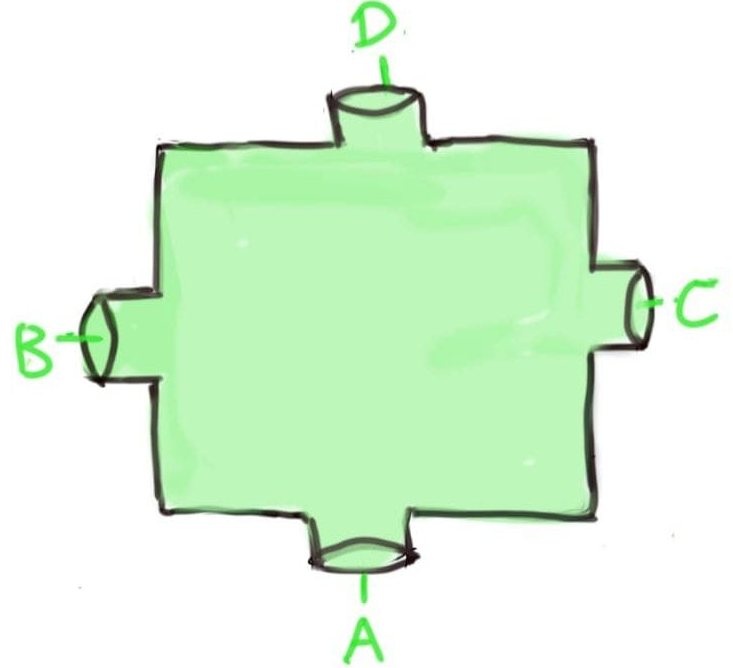Hydraulic Machines

Consider a vessel having four openings A, B, C and D, these openings are provided with frictionless, weightless and watertight pistons. All the four openings are equally wide and having a cross-section area a.

When the piston at A is pushed in with a force F, it exerts a pressure P = F/a on the enclosed fluid. It is found that we require the same force F on each piston B, C and D to keep them from moving.

Thus, it proves that the external pressure applied to enclose fluid is transferred to all points undiminished.

### Hydraulic Lift

Hydraulic Lift is mainly used to lift loads by applying a force of lesser magnitude. A simple hydraulic lift consists of two cylinders 1 and 2 of different cross-section areas A1 and A2 connected together with the horizontal pipe. Two cylinders are provided with frictionless airtight pistons. An incompressible oil is filled in the cylinders.

One piston has a smaller area of cross-section than the other. Suppose we push piston 1 down with a force F1. Therefore the pressure exerted in this piston is,

P1 = F1/A1

Now according to Pascal’s law, this change in pressure is transferred undiminished to the other piston P2.

ΔP1 = ΔP2

F1/A1 = F2/A2

or

F2 = F1 × A2/A1

A2 >> A1

Thus, force F2 experienced by piston 2 is greater than the applied force F1, by the factor A2/A1.

Hence, by applying a small force at the narrower piston we can balance a greater force (weight of the car) at the wider piston. Thus, effectively by using a hydraulic lift, a given force applied over a given distance can be transformed to a greater force applied over a smaller distance.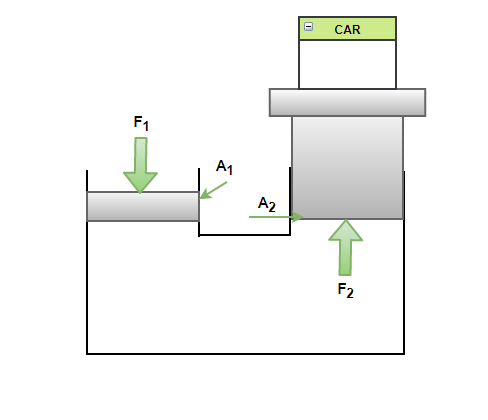Hydraulic Lift

### Hydraulic Brakes

Hydraulic brakes in automobiles work on Pascal’s principle of transmission of fluid pressure. In a simple arrangement when the vehicle driver applies force on the brake pedal with his foot, the lever system moves the piston inside a master cylinder containing break oil. The pressure caused is transmitted through the brake oil to pistons P1 and P2 of a larger area. A larger force acts on the pistons and pushes the brake shoes against the brake lining. In this way, a small force on the brake pedal produces a large retarding force on the moving wheel. When the brake pedal is released, the spring pulls the brake shoes to their normal positions. Thus, forcing brake oil to flow back to the master cylinder.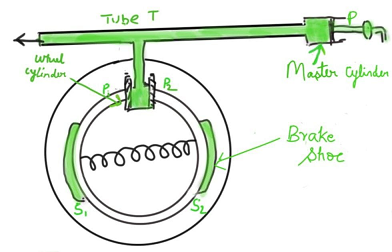When a little force is applied on the pedal with the foot, the master piston moves inside. The pressure thus transmitted is transferred undiminished to the other pistons P1 and P2 which are directly connected to the wheels. These pistons are of larger cross-sections as compared to the master piston P.

Applying Pascal’s law, a large force acts on these pistons that move out and expand the brake shoes. Thus, the braking force produced at the wheels is quite large as compared to the little force applied at the brake pedal. The breaking effort is equal in all the wheels because the pressure is transferred equally to all the pistons connected to the wheels.

### Sample Questions

Question 1: What is the pressure on a swimmer 10 m below the surface of a lake?

Given that, the height, h = 10 m.

ρ ( water ) = 1000 kg/m3 , g = 9.8 m/s2

Pressure on the swimmer 10 m below the surface of the lake,

P = Pa + h*ρ*g

= 1.0 * 105 + 10*1000*9.8

= 1.98 * 105 Pa  ≈ 2 atm

Question 2: The density of the atmosphere at the sea level is 1.29 kg/m3. Assume that it does not change with altitude. Then how high would the atmosphere extend? (Take g = 9.81 m/s2).

Here ρ = 1.29 kg/m3 , g = 9.81 m/s2 , Pa = 1.01 * 105 Pa

As, Pa =  h × ρ × g

h = Pa /  ρ × g

= 1.01  ×  105 / 1.29  ×  9.81

= 7981 m  ≈ 8 km

Question 3: Reading of mercury level in a barometer installed in an establishment in the hilly terrain on a day was found to be 732 mm. Find the value of atmospheric pressure at the place. Given that density of mercury is 13.6*103 kg m-3 and g =9.8 m s-2

Given that, h = 0.732 mm = 0.732m,

ρ = 13.6 × 103 kg m-3 and

g =9.8 m s-2

Atmospheric pressure at the place,

Pa = h × ρ × g = 0.732 × 13.6 × 10 × 9.8

= 9.76 × 104 Pa.

Question 4: The pistons of a hydraulic machine have diameters of 30.0 cm and 2.5 cm. What is the force exerted on the larger piston when 40 kg wt. is placed on the smaller piston? If the smaller piston moves in through 6 cm, how much does the other piston move out?

For smaller piston , Area a1 = π × (1.25)2 cm2

For larger piston   ,Area a2 =  π × (15)2 cm2

Mechanical advantage at the larger piston is a2/a1

F2  = F1 × a2/a1 = 40 × π × (15)2/ π (1.25)2

= 40 × 9.8 × 225/1.25 × 1.25

= 56,448 N

This is the force exerted on the larger piston. The liquids are considered incompressible. Therefore volume covered by the movement of the smaller piston inwards is equal to that moved outwards by larger piston.

l1a1 = l2a2

l2 =l1 × a1/a2 = 6  × (1.25)2/(15)2 cm

= 0.042 cm

So, the distance moved out by the larger piston is 0.042 cm.

Question 5: Water is filled in a flask up to a height of 40 cm, the bottom of the flask is square with side 10 cm. If the atmospheric pressure is 1.01 × 105 N/m2, find the force exerted by water on the bottom. Take g = 10 m/s2 and square density of water = 1000 kg/m3

The pressure at the surface of water is equal to the atmospheric pressure P0 . The pressure at the bottom is

P = P0 + hρg

= 1.01 × 105 + 0.40 × 1000 × 10

= 1.01 × 105 + 0.04 × 105

= 1.05 × 105 /m2

The area of the bottom = a2 = (10 × 10-2)2 = 102 m2

The force at the bottom = F = P  ×  area of bottom

= (1.05 × 105)  ×  (10-2)

= 1.5  ×  103 N

The force on the bottom is 1050 N.

Question 6: A barometer kept in an elevator accelerating upwards reads 76 cm of Hg. If the elevator is accelerating upwards at 4.9 ms-2, What will be the air pressure in the elevator?

When the elevator moves upwards with acceleration a.

Net acceleration = g + a

Thus, Pressure = h × ρ × (g + a) dyne cm-2

= 76 × 13.6 × (9.8 + 4.9) / 13,6 × 9.8

P = 114 cm of Hg

My Personal Notes arrow_drop_up
Related Tutorials